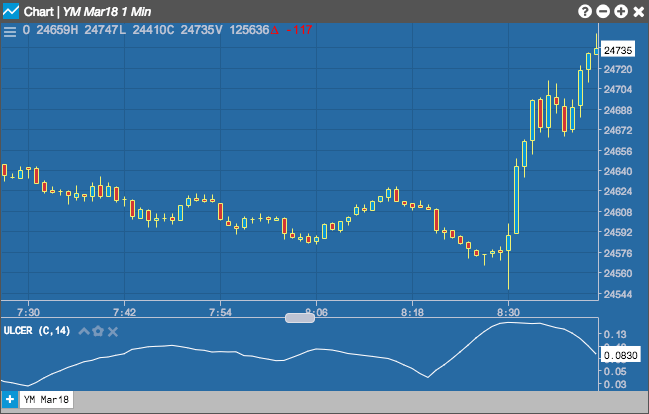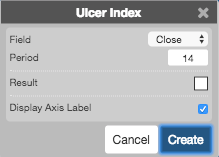Charts

# Ulcer Index

The Ulcer Index (UI) indicator can be used to determine downside risk by displaying the depth and duration of price declines from their earlier highs. A high UI value is generally an indication that the instrument's price has moved further away from its previous high and may take longer to return to this value.## Configuration Options• Field: Price or combination of prices to use as the base for average calculations. Possible values include:
• Open
• High
• Low
• Close
• HL/2 $$\left ( \frac{High + Low}{2} \right )$$
• HLC/3 $$\left ( \frac{High + Low + Close}{3} \right )$$
• HLCC/4 $$\left ( \frac{High + Low + Close + Close}{4} \right )$$
• OHLC/4 $$\left ( \frac{Open + High + Low + Close}{4} \right )$$
• Period: Number of bars to use in the calculations.
• Color Selectors: Colors to use for graph elements.
• Display Axis Label: Whether to display the most recent value on the Y axis.

## Formula

The indicator is calculated in three steps:

$Percentage\; Drawdown = \frac{Close - (n\text{-}period\; High\; Close)}{n\text{-}period\; High\; Close} \times 100$

$Squared\;Average = \frac{\sum_{n=1}^{n\text{-}period} (Percentage\;Drawdown)^2}{n\text{-}period}$

$Ulcer\;Index = \sqrt{Squared\;Average}$